# What Is a Triangular Number?

Ms. Inglish provided the largest numbers of graduations in alternative high school STEM programs in Ohio for 10 years.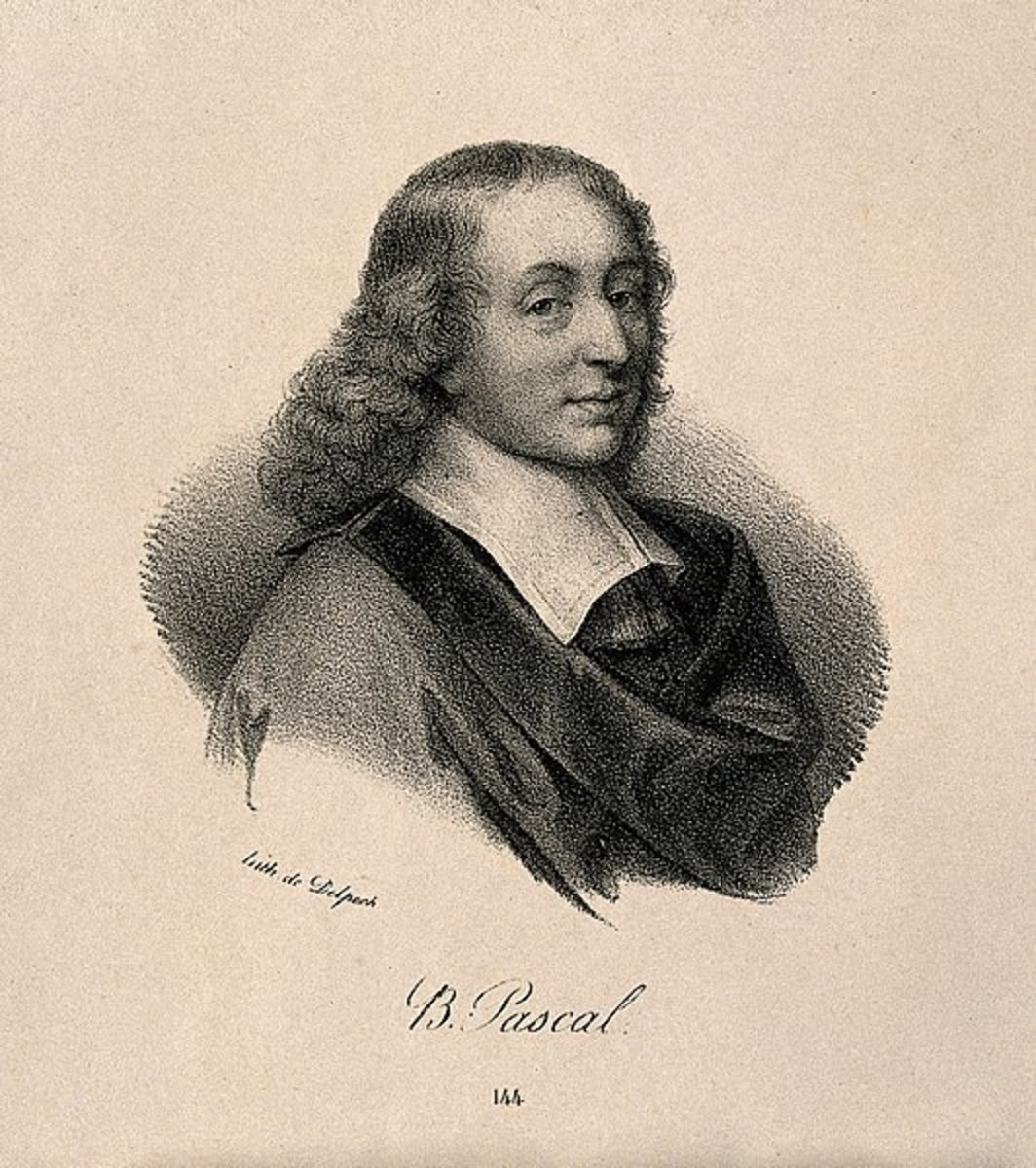Blaise Pascal developed a triangle with useful "magical" properties, but ancient Asians had already developed such a figure.See page for author [CC BY 2.0 (http://creativecommons.org/licenses/by/2.0)], via Wikimedia Commons

## A Mathematical Problem of Intersections

This mathematical question was asked of Hubbers:

Fibonacci numbers are numbers of the form:

1,1, 2, 3, 5, 8, 13,21, 34, 55,89...x, y, x+y.......

Triangular numbers are numbers of the form:

1, 3, 6, 10, 15, 21, 28, 36, 45, 55, 66...

Now my question: Using an equation can we show a relationship between Fibonacci Numbers and Triangular Numbers? If yes, what is it?

The short answer is that there is an intersection of the two formulas that yields Pascal's Triangle.

How do we derive a formula for Pascal's Triangle?

I think there is probably at least one intersection that can be found between the two number sequences in the question that would aide in gaining a formula. Finding the formula is rather complex.

When thinking about this dilemma for the first time, one might see images of intersections of conic sections or a straight addition of two formulas to find a crossing-point, or even some sort of fractal arrangement. It can be a complex matter in which an investigator gets in his own way during the derivation of a formula. However, with enough time to think about the problem, the exercise can be entertaining.

### Triangles and Quadrilaterals

This is fun for individuals that like number puzzles and/or geometry.

• Triangular numbers are made up of equilateral triangular arrangements and
• Fibonacci numbers are built on spiraled squares and rectangles.

Since equilateral triangles can fit into squares and rectangles in certain instances as to fill up the area of those quadrilaterals, there would seem to be a relationship between the 3- and 4-sided figures.

An intuitive assumption is that if some number and size of equilateral triangles can fit completely into the specific squares and rectangles observed, then a relationship between triangular numbers and Fibonacci numbers exists.

Some equilateral triangles may need to be bisected and the two parts realigned around another triangle for this to be so in some cases. Some other division of the triangles may be necessary in other cases. We see this type of problem in books of puzzles frequently.

The formula for triangular numbers is present in a variety of text books:

## TABLE I. Examples of Triangular Numbers

n (n+1)/2

1

1(2)/2

1

2

2(3)/2

3

3

3(4)/2

6

4

4(5)/2

10

5

5(6)/2

15

6

6(7)/2

21

7

7(8)/2

28

8

8(9)/2

36

9

9(10)/2

45

10

10(11)/2

55

20

20(21)/2

210

Graphically, a series of triangular numbers looks like a line of two-dimensional pyramids becoming larger when read from left to right.

For fun, let's look at a graph of the triangular numbers themselves.

## GRAPH I. Triangular Numbers Graph from TABLE I.

Scroll to Continue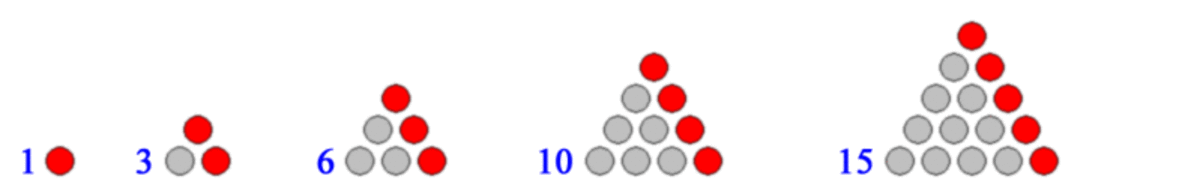By Aldoaldoz (Own work) [CC BY-SA 3.0 (http://creativecommons.org/licenses/by-sa/3.0) or GFDL (http://www.gnu.org/copyleft/fdl.html)], via Wikimedia C

### Fibonacci Sequence Numbers and Formula

Every Fibonacci Number in this series is the sum of the preceding two numbers. This formula is also logged in many mathematics texts and handbooks:

Fn = Fn-1 + Fn-2

or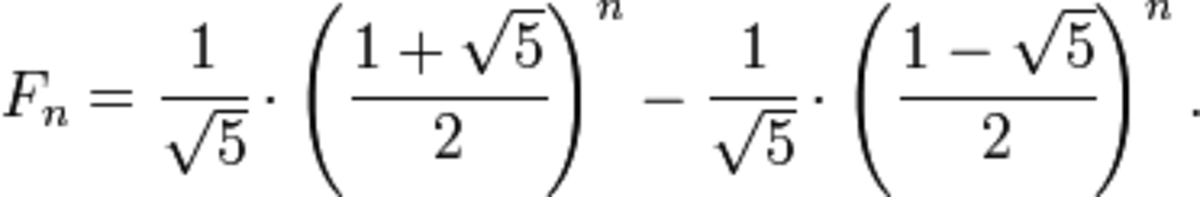Binet's Formula for the "nth" Fibonacci Number in a Sequence

## To easily find the "nth" number in a Fibonacci Sequence, use this online calculator.

• Fibonacci Calculator
Fibonacci numbers and the golden section calculator with multi-precision arithmetic. Also powers of Phi and the Rabbit sequence.

## TABLE II. A Fibonacci Series

Sum of Two Previous

1

1

2

3

5

8

13

21

34

## GRAPH II. Graph of Fibonnacci Numbers from TABLE II.

A sequence of Fibonacci Numbers ultimately leads to a spiral(ing) shape, one that encompasses a fractal equation(s). Enjoy the animation and the video below for more answers.

## Musical Fibonacci Sequence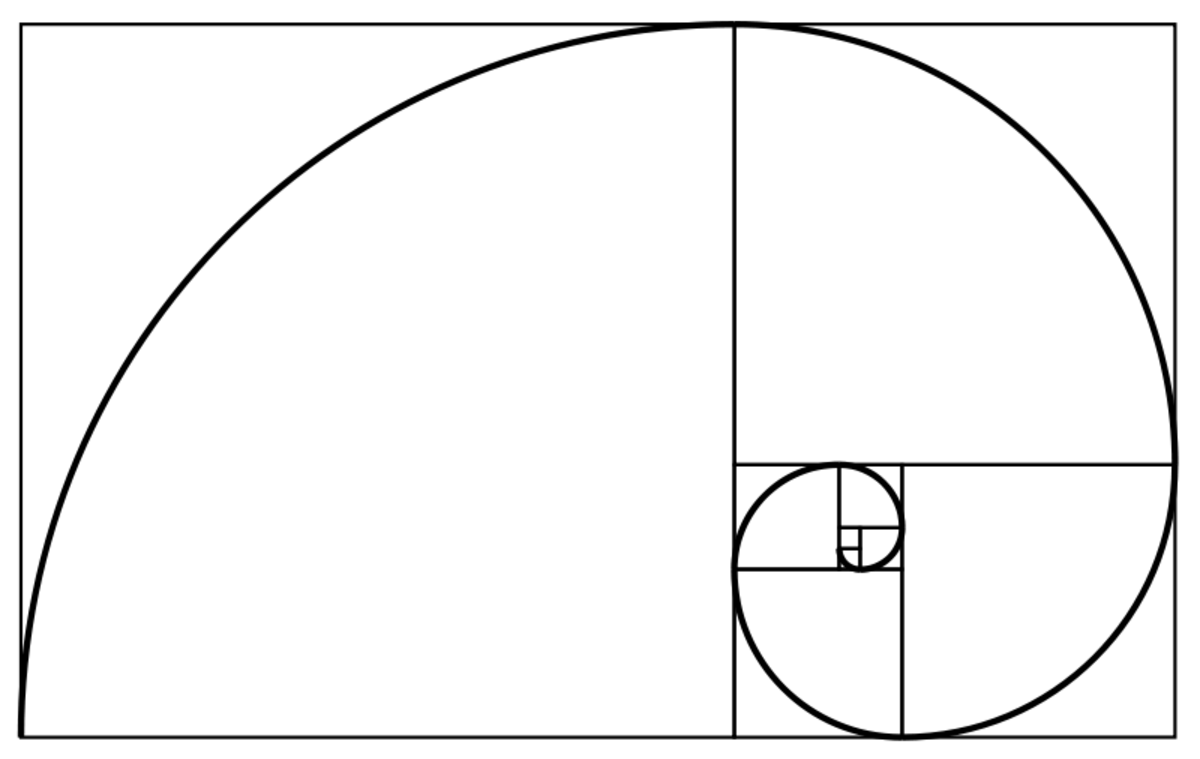A Fibonacci Spiral. NOTICE THE SQUARES IN THE PATTERN AROUND THE SPIRAL.

### Experiment With Numbers

As an experiment, we might try simply adding together the two equations - adding the resulting points together. This will likely end up to be a nonsense curve when graphed. Nevertheless, someone will try it to see what it looks like, so let's do it here:

TFibonacciResult

3

1

4

6

1

7

10

2

12

15

3

18

21

5

26

28

8

36

36

13

49

45

21

66

## GRAPH III. Experimental Graph From TABLE III.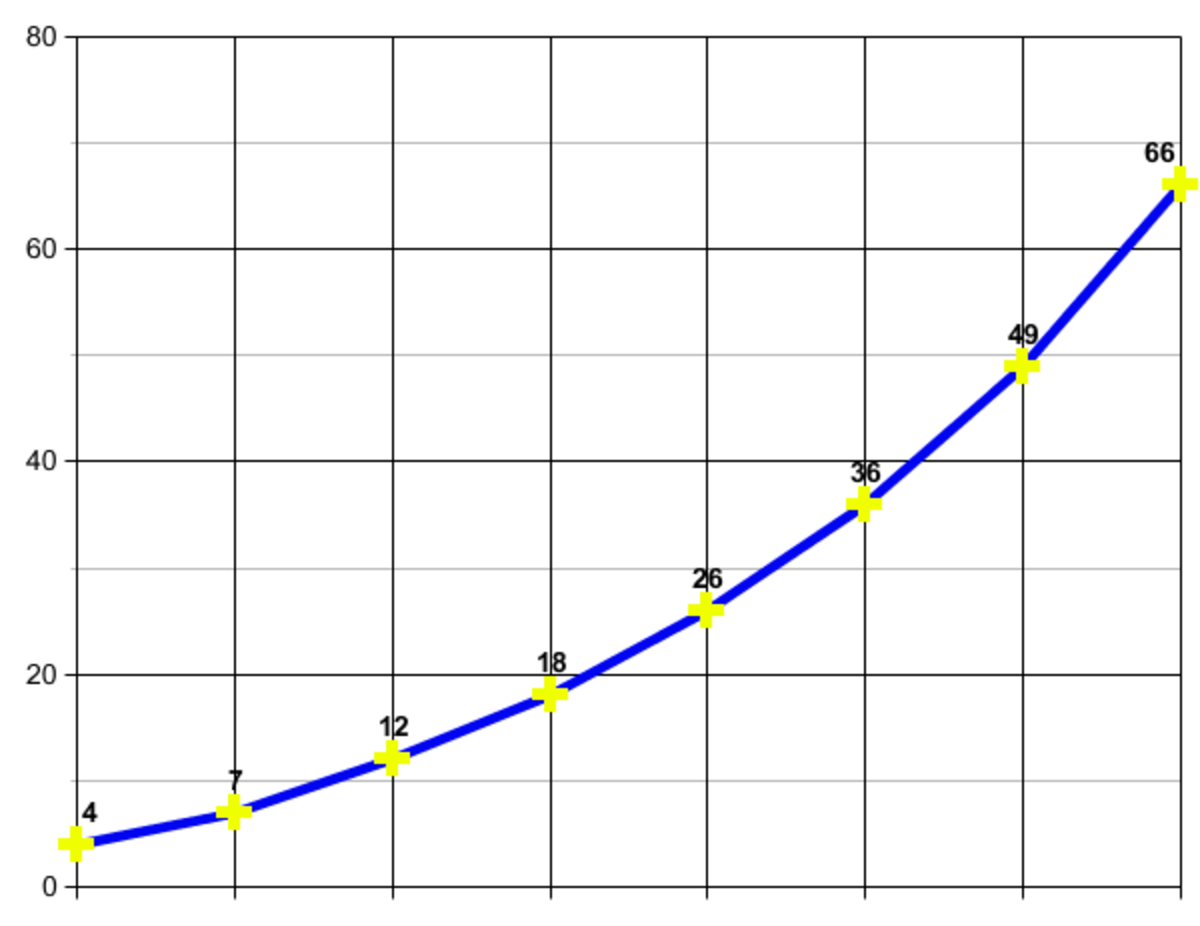Surprisingly, we achieve a fairly clean curve, although not very useful.(c) Patty Inglish 2011

The other thing to try is to add the formulas for nth Triangular Number and nth Fibonacci Number. This is quite tedious. but can be done.

(n2 + n)/2

plus, rounding the square root of 5 to 2.24 in Binet's Formula we can simplify:

(5.24n - 0.77n)/2.24

So the addition of formulas is:

(n2 + n)/2 + (5.24n - 0.77n)/2.24

nTFResult

0

0.0

0.0

0.0

1

0.5

2.03

2.53

2

3.0

12.02

15.02

3

6.0

64.03

67.03

4

10

336.45

345.45

## GRAPH IV. Graph from TABLE IV.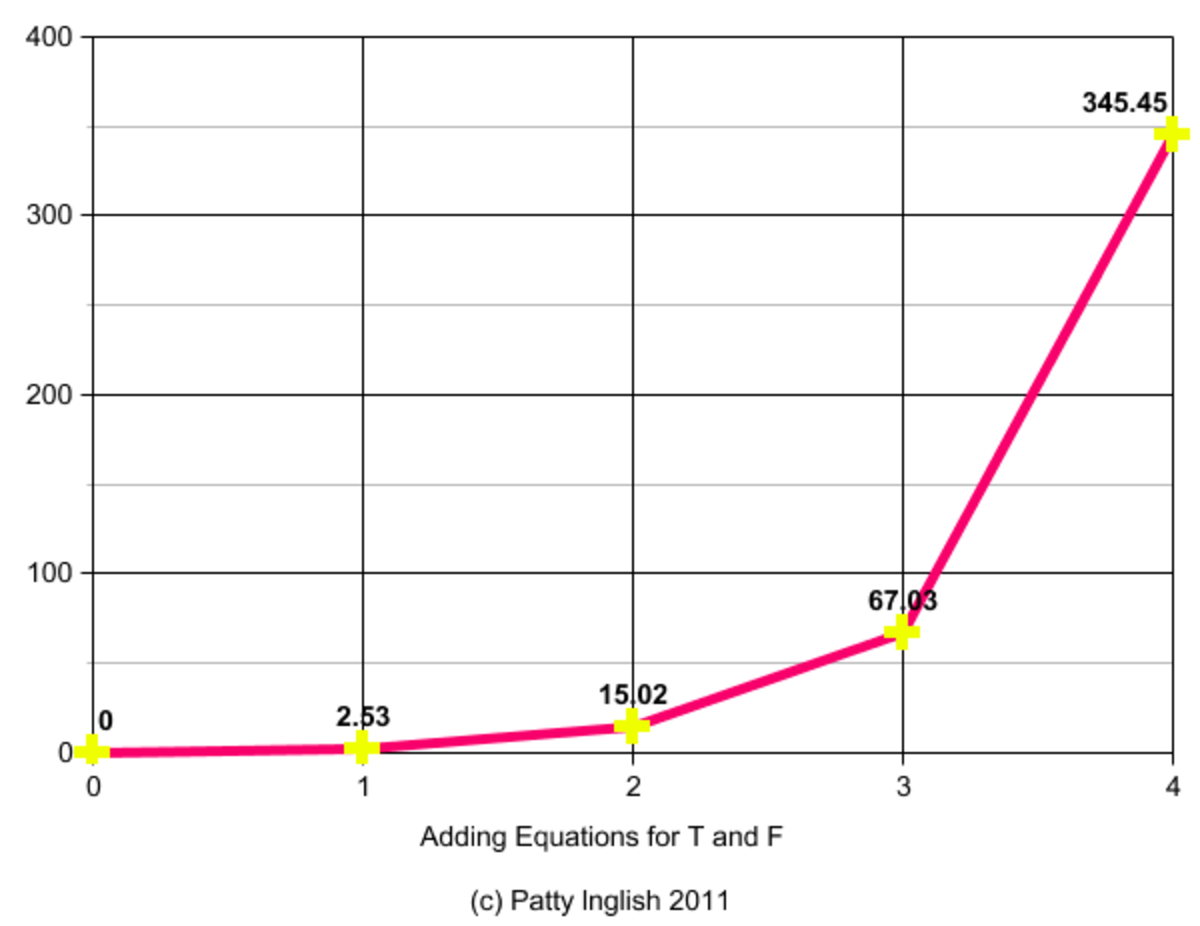This line looks more of an attempt at a spiral entity.

## Asian Developments Before Blaise Pascal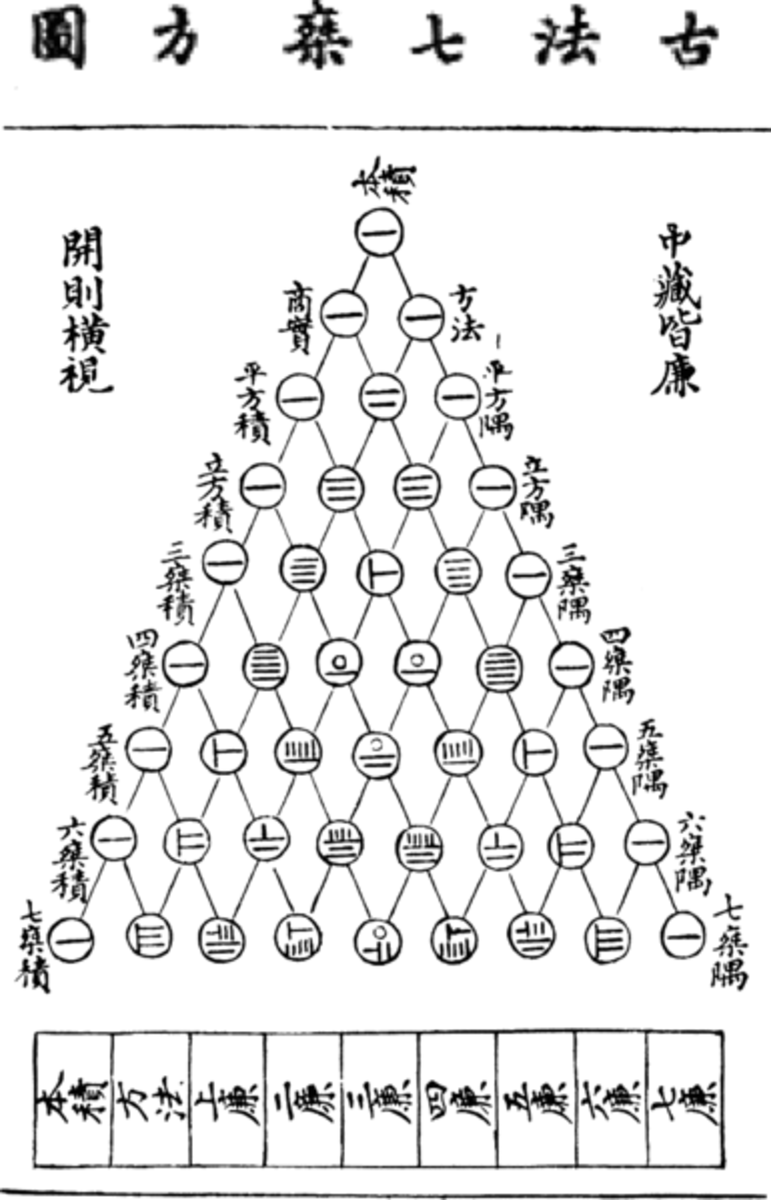Before Pascal's Triangle. The Yanghui Triangle of mathematician Yang Hui. It was called a chart of Seven Multiplying Squares.public domain

The above diagram is a triangle from Ancient China that was used to assist in finding cube roots of numbers. It is Pascal's Triangle before Blaise Pascal discovered its formula in the 13th century AD.

.

The triangle, also found in other ancient societies like Japan, Persia, and India, is an instance of an intersection of or relationship between triangular numbers and Fibonacci numbers.

### A Triangle Calculator

A the end of experiments and derivations, mathematics has found that a relationship between triangular numbers and Fibonacci numbers exists in Pascal's Triangle.

The information below reveals construction, formulas, and associated uses for the specialized triangle that is like a calculator.

### A Pascal's Triangle Online Calculator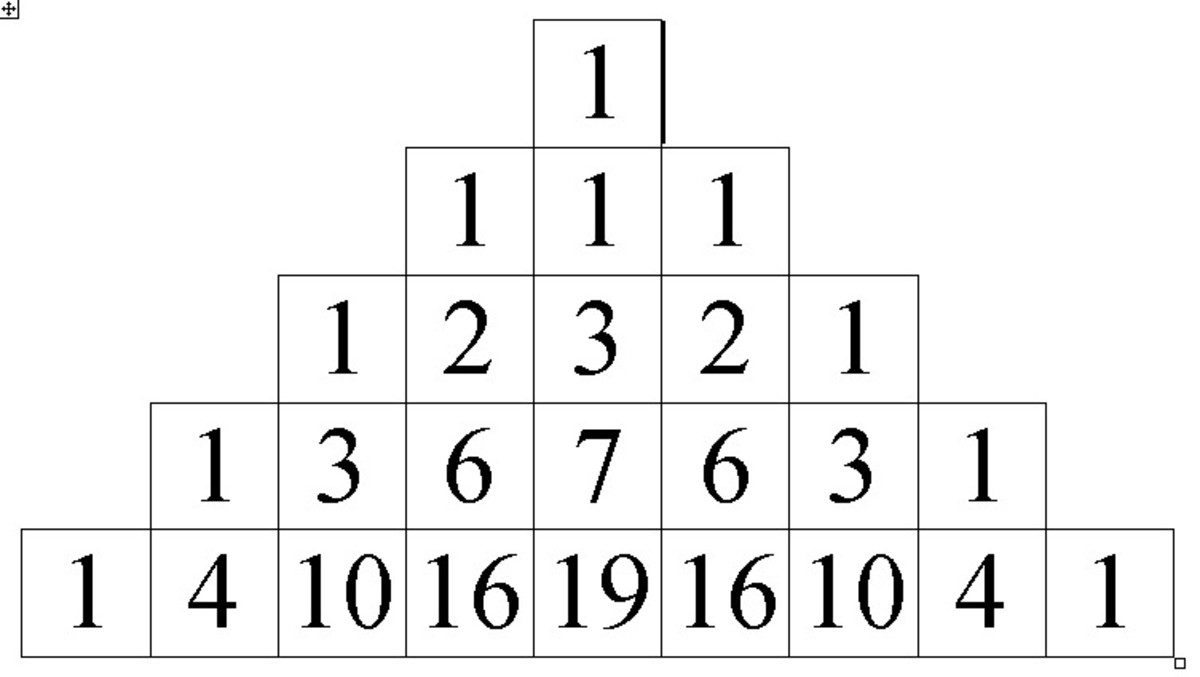A Pascal's Triangle: Each number in the triangle is the sum of two above.public domain

Triangular numbers are contained within Pascal's Triangle. Start with the first "1" in the second row from the top of the triangle and look diagonally down through a line moving to the southeast. The larger the triangle you create, the greater the number of triangular numbers you will be able to read.

Pascal's Triangle can be used for probabilities and for other calculations (see videos below).

### Use Pascal's Triangle to Figure Binomial Coefficient: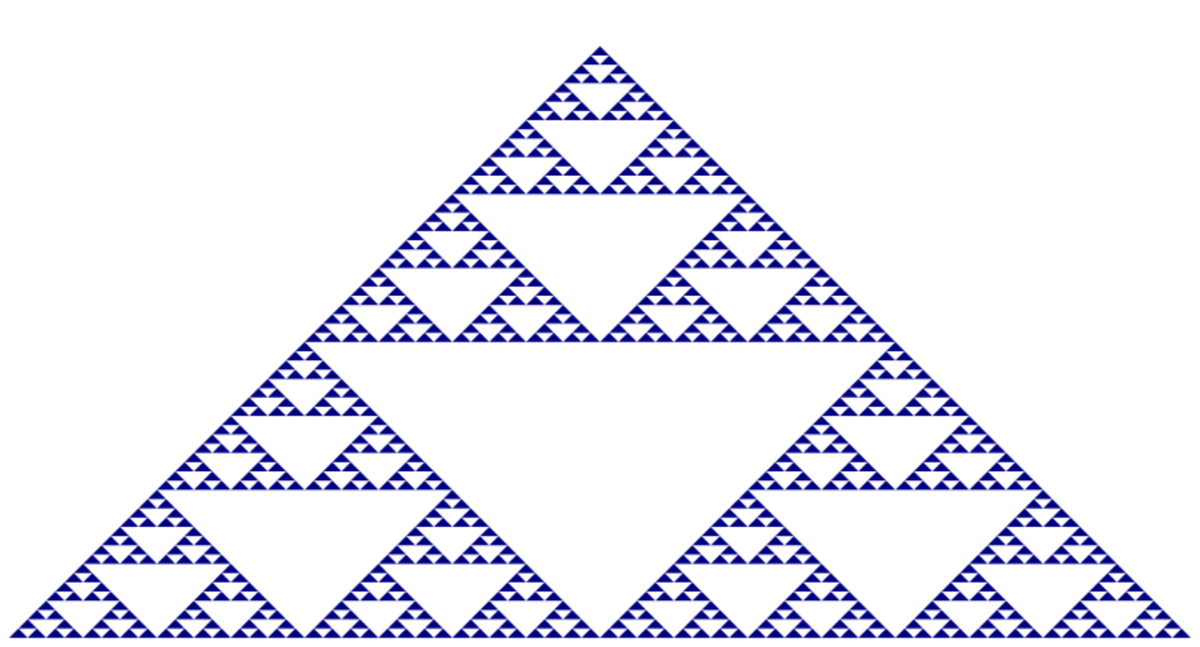A Fractal Pattern within Pascal's Triangle. This pattern is often used in making quilt patterns.public domain

## More on Mathematics

This content is accurate and true to the best of the author’s knowledge and is not meant to substitute for formal and individualized advice from a qualified professional.

© 2011 Patty Inglish MS

Patty Inglish MS (author) from USA and Asgardia, the First Space Nation on October 31, 2011:

Man from Meodesto - ~exactly... :)

Pemekwulu - Mathmatics contians music, art and poetry, of course.

pemekwulu from USA on October 31, 2011:

Amazing! This is very interesting and informative.This hub helps to bring out the beauty, harmony, elegance, aesthetics inherent in mathematics. Number theory is an amazing field of study.

Keep up the good work.

Man from Modesto from Kiev, Ukraine (formerly Modesto, California) on October 30, 2011:

2 + 2 = 5

... for very large values of 2.

Patty Inglish MS (author) from USA and Asgardia, the First Space Nation on May 03, 2011:

Thanks for contents - I hoped it would be entertaining as well.

Hello, hello - I really like the theory portrayed in the flowers and sea shells. Nature is amazing!

Hello, hello, from London, UK on May 03, 2011:

Hello Patty, this is way above me, I only had elemantary schooling and commercial college.

pemekwulu from USA on April 30, 2011:

Amazing hub. I had wanted two mathematical expressions A and B that use Fibonacci numbers as inputs to generate even-subscripted and odd-subscripted triangular numbers respectively.

Deya Writes on April 27, 2011:

I remember Pascal's Triangle...probably one of the most useful tools I have ever remembered in math. (Besides the trigonometric circle and all its bits and pieces) Great hub! Voted UP :)

Dim Flaxenwick from Great Britain on April 26, 2011:

Well, l`pressed up and awesome. because your hubs always are, but l´m afraid l was lost before halfway down the page... Just not my area, l think.

Simone Haruko Smith from San Francisco on April 26, 2011:

Oh, wow! I'm rather averse to math, but I loved this Hub! Very well explained and illustrated!

Patty Inglish MS (author) from USA and Asgardia, the First Space Nation on April 26, 2011:

A secret lab of mathematicians in an underground bunker...

Number is theory is fun when you some leisurely time to look at it - and the tools to make graphics, even if some have little or no meaning. It helps brings things together and show processes. Kind of like a mystery novel - or absurdist theater.

Thanks for all the comments!

kafsoa on April 26, 2011:

Great way to simplify concept.this is a real model hub!

Mike Teddleton from Midwest USA on April 26, 2011:

Fun stuff, outstanding answer to the question What Is a Triangular Number?

Thanks for all the information. Mike

BlissfulWriter on April 26, 2011:

That's very interesting. I always love math.

Earth Angel on April 26, 2011:

Dearest Patty,

Ahhhhhh, now I know how you produce all these amazing Hubs! You have an entire staff of brilliant and genius researchers which you pay millions!! ;-)

I'm not even particularly fond of numbers/mathematics and you had me reading each and every entry with fascination and amazement!

This Hub is its own Fibonacci Spiral! And you are the amazing Intersection of all!

Blessings always, Earth Angel!

Sun Pen 50 from Srilanka on April 26, 2011:

Interesting Hub. Thank you for sharing.

The Old Firm from Waikato/Bay Of Plenty, New Zealand on April 25, 2011:

Hi Patty,an interesting article.

Although I was unaware of them being triangular numbers, I use them to calculate the total combinations I have to make to cover all of a selection of numbers for lotto, (or horses in a race) An exercise, I'm not much of a gambler.

As an example, if I have to combine 5 numbers of 8 non sequentially every possible way, the action goes: 1+2+3+4+5, 1+2+3+4+6, 1+2+3+4+7, 1+2+3+4+8, (total 4) 1+3+4+5+6, 1+3+4+5+7, 1+3+4+5+8 (total 3) 1+4+5+6+7, 1+4+5+6+8 (total 2) and 1+5+6+7+8 (total 1) giving a sub total for the 1 series of 4+3+2+1=10 combinations. Dropping the 1 and doing the 2 sequence gives 10-4=6 combinations the next 6-3=3,and the4 sequence 3-2=1 (4+5+6+7+8, see told you so!)

So the total combo's are 10+6+3+1=20. Does this sequence look familiar? yep, triangular.

It's a lot easier and quicker to do than the tedious explanation, but I'll give that bit a miss. Even the short-cut is a pain to explain, thanks to my convoluted mathematical brain.

Cheers

Peter.